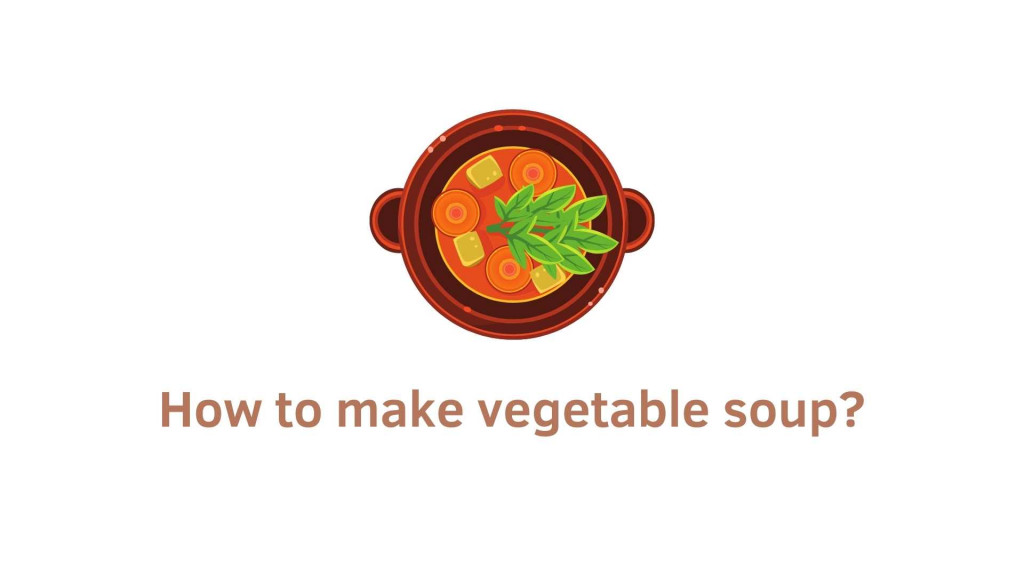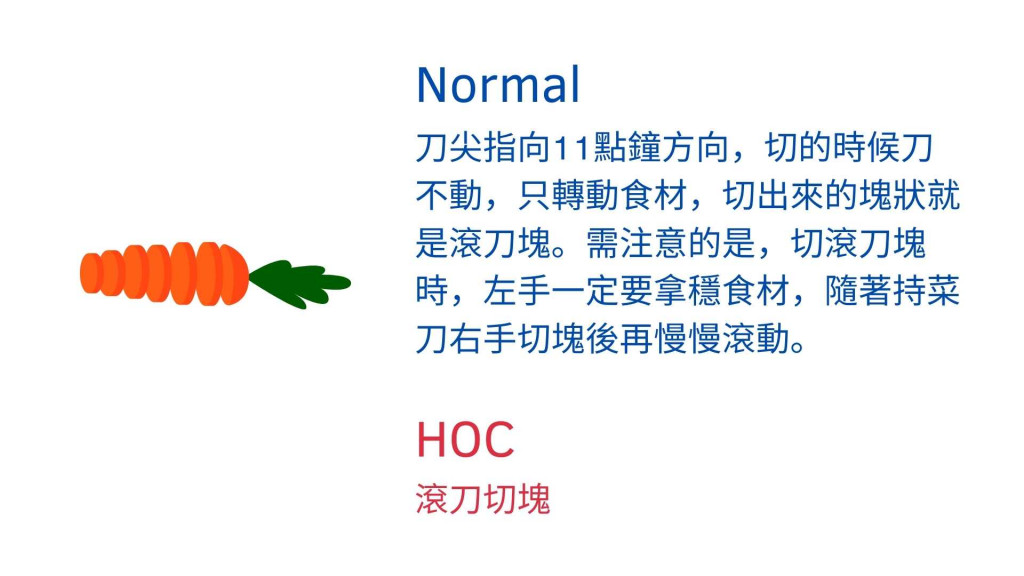#DAY 11
3
Software Development

## Higher-order functionFP 原則就是把 Function 作抽象化並極小化，Higher-order function 也就是抽象函式的一種，抽象式內容不包含執行步驟的細節，而是在 high level 的角度描述解決方法，例如以下有兩種煮蔬菜湯的描述

### 2. 抽象思維``````// 1)
let total = 0, count = 1;
while (count <= 10) {
total += count;
count += 1;
}
console.log(total);
``````
``````// 2)
console.log(sum(range(1, 10)));
``````

# First-class function

javaScript 的 function 是 first-class citizens，所以我們可以

#### 3. Return functions from other functions

``````/* 1. Assign functions to variables ---- */
const fisrtClassFunction1 = function() {}

/* 2. Pass functions as arguments to other functions --- */
const fisrtClassFunction2 = **callback** => {
console.log("HOF...");
callback();
}

function callbackFunction () {
console.log("callbackFunction...");
}

// 除了 number、string、object、array 等等可以當參數外，
// function 也可以當成參數傳入 function
fisrtClassFunction2(callbackFunction);

/* 3. Return functions from other functions ---- */
const fisrtClassFunction3 = function() {
return function() {
console.log("callbackFunction...");
}
}
const myFistClassFunction = fisrtClassFunction3(); // return a function
myFistClassFunction();
``````

``````// use ES6 arrow function instead
const fisrtClassFunction3 = () => () => {
console.log("callbackFunction...");
}

const myFistClassFunction = fisrtClassFunction3(); // return a function
myFistClassFunction();
``````

# Higher-order function (HOF)

A function that takes a function as an argument, or returns a function as a result
wiki

#### 1. Takes one or more functions as arguments

``````// 把 x => x + 1 函式當作參數傳入 map 函式
[1,2,3].map(x => x + 1); // => [2, 3, 4]
``````

## 來看一個簡單的例子吧

``````for (let i = 0; i < 10; i++) {
console.log(i);
}
``````

``````function repeatLog(n) {
for (let i = 0; i < n; i++) {
console.log(i);
}
}
``````

``````function repeat(n, action) {
for (let i = 0; i < n; i++) {
action(i);
}
}

repeat(3, console.log);
// → 0
// → 1
// → 2
``````

``````let labels = [];
repeat(5, i => {
labels.push(`Unit \${i + 1}`);
});
console.log(labels);
// → ["Unit 1", "Unit 2", "Unit 3", "Unit 4", "Unit 5"]
``````

# Higher-order functions 實務上的用途

### Curried function / Composition

#### 參考文章

``````如有錯誤或需要改進的地方，拜託跟我說。

``````